Скачать презентацию Maths at Te Kowhai School Information for Parents

865db4ed7a65672949a9be243b7f5d79.ppt

• Количество слайдов: 35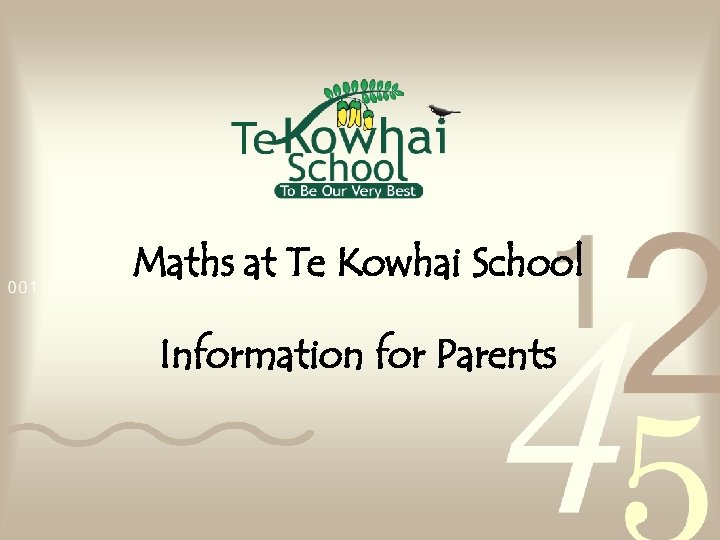Maths at Te Kowhai School Information for Parents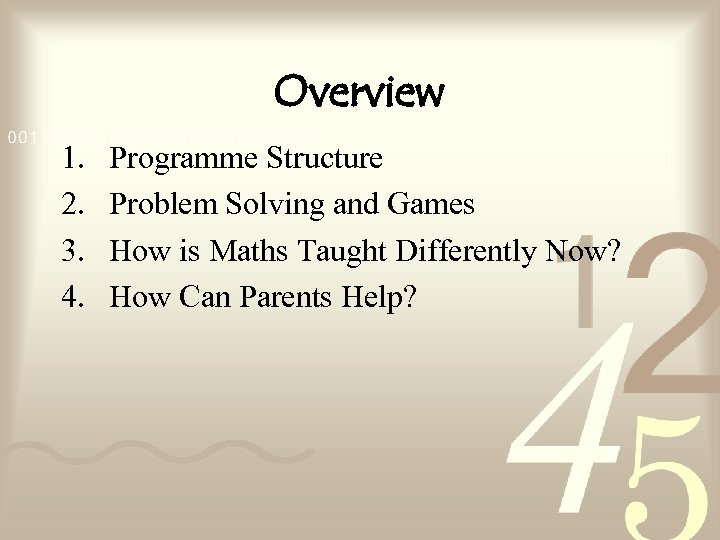Overview 1. 2. 3. 4. Programme Structure Problem Solving and Games How is Maths Taught Differently Now? How Can Parents Help?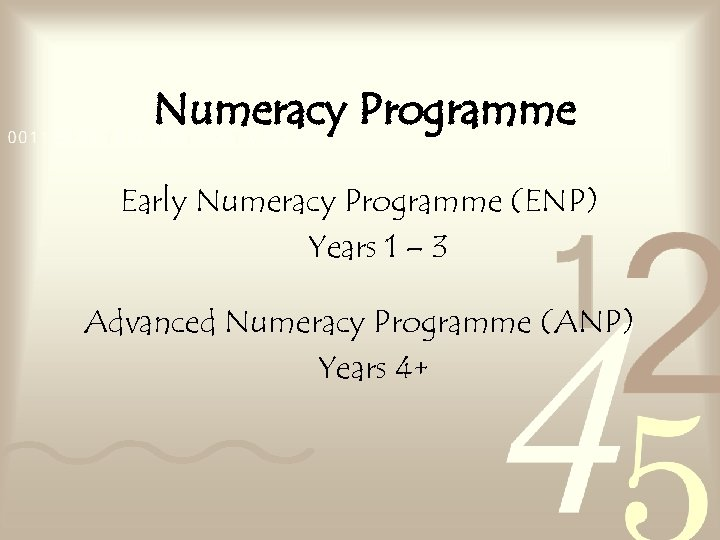Numeracy Programme Early Numeracy Programme (ENP) Years 1 – 3 Advanced Numeracy Programme (ANP) Years 4+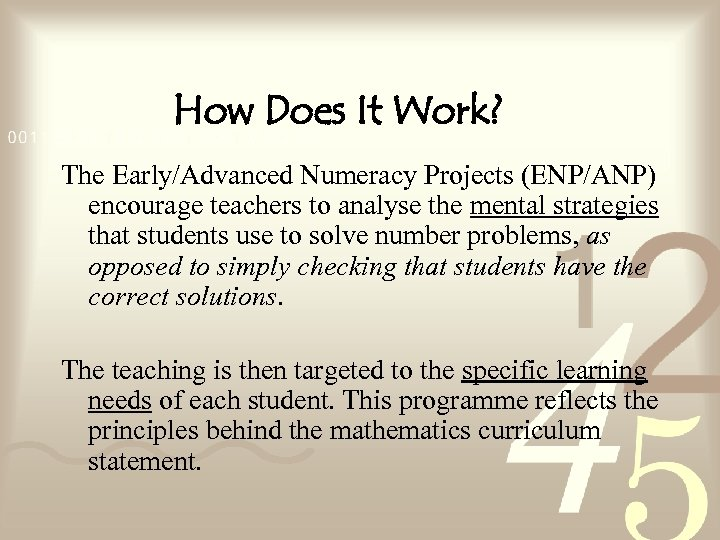How Does It Work? The Early/Advanced Numeracy Projects (ENP/ANP) encourage teachers to analyse the mental strategies that students use to solve number problems, as opposed to simply checking that students have the correct solutions. The teaching is then targeted to the specific learning needs of each student. This programme reflects the principles behind the mathematics curriculum statement.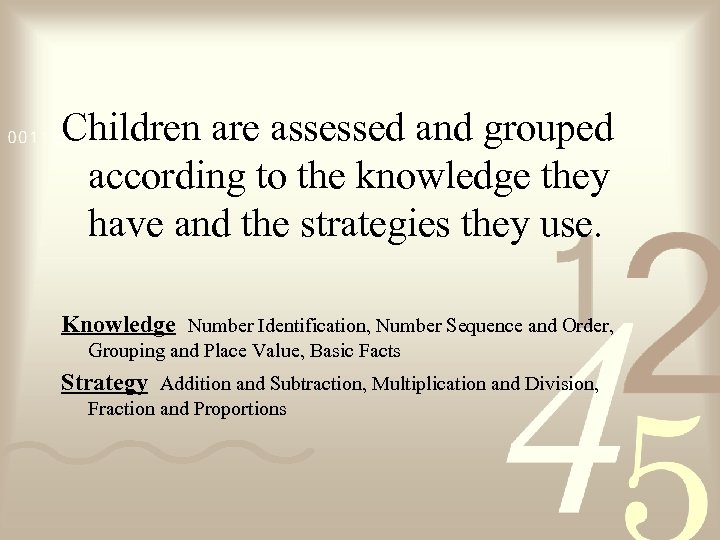Children are assessed and grouped according to the knowledge they have and the strategies they use. Knowledge Number Identification, Number Sequence and Order, Grouping and Place Value, Basic Facts Strategy Addition and Subtraction, Multiplication and Division, Fraction and Proportions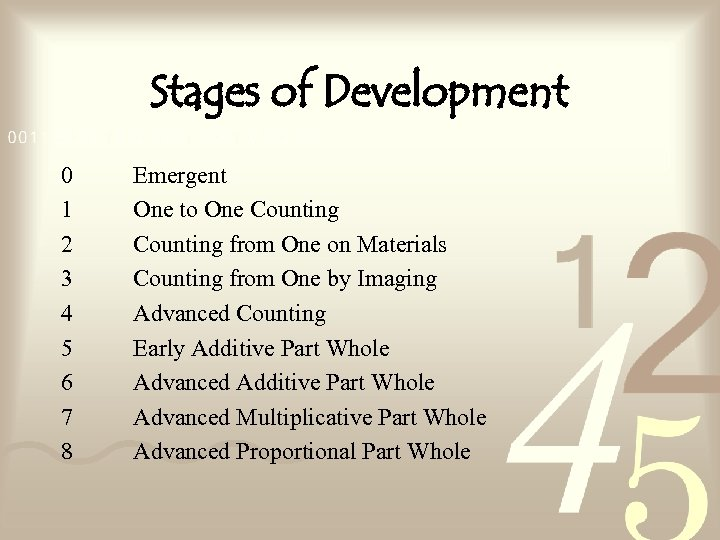Stages of Development 0 1 2 3 4 5 6 7 8 Emergent One to One Counting from One on Materials Counting from One by Imaging Advanced Counting Early Additive Part Whole Advanced Multiplicative Part Whole Advanced Proportional Part Whole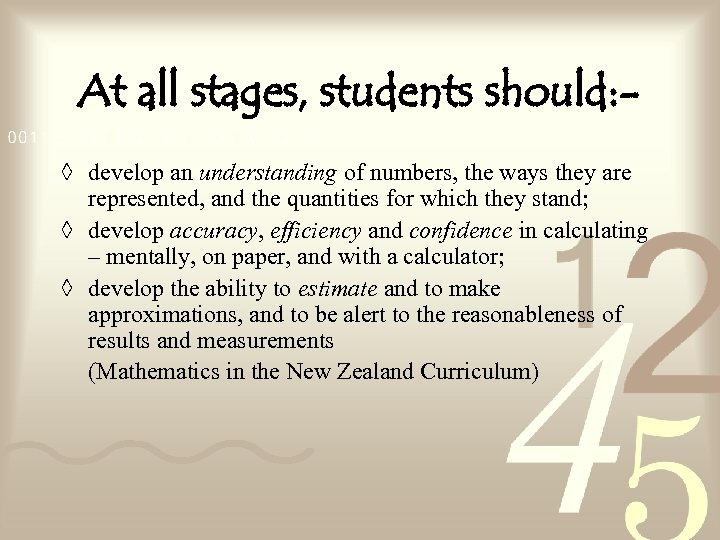At all stages, students should: ◊ develop an understanding of numbers, the ways they are represented, and the quantities for which they stand; ◊ develop accuracy, efficiency and confidence in calculating – mentally, on paper, and with a calculator; ◊ develop the ability to estimate and to make approximations, and to be alert to the reasonableness of results and measurements (Mathematics in the New Zealand Curriculum)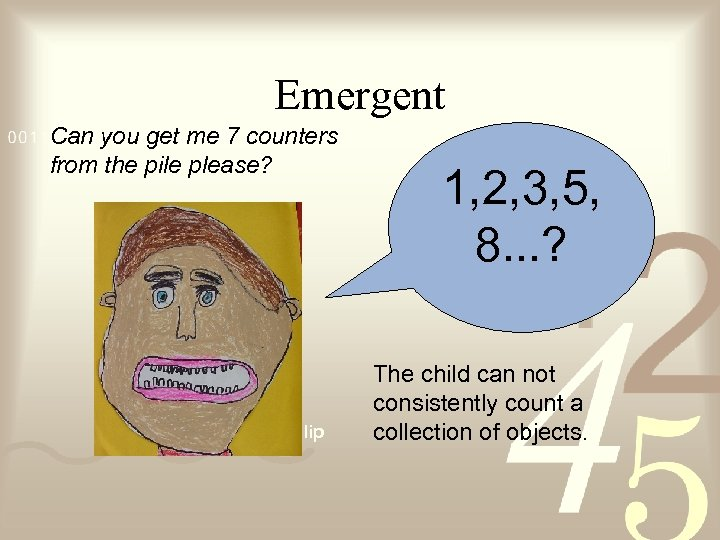Emergent Can you get me 7 counters from the pile please? Movie Clip 1, 2, 3, 5, 8. . . ? The child can not consistently count a collection of objects.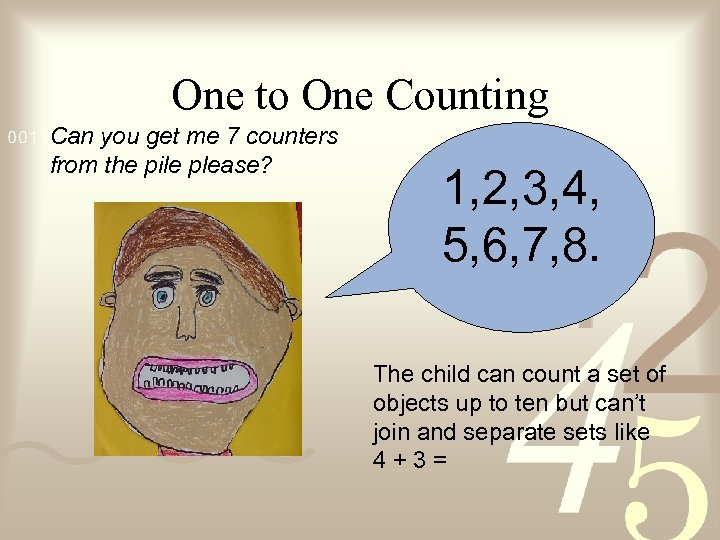One to One Counting Can you get me 7 counters from the pile please? 1, 2, 3, 4, 5, 6, 7, 8. The child can count a set of objects up to ten but can’t join and separate sets like 4+3=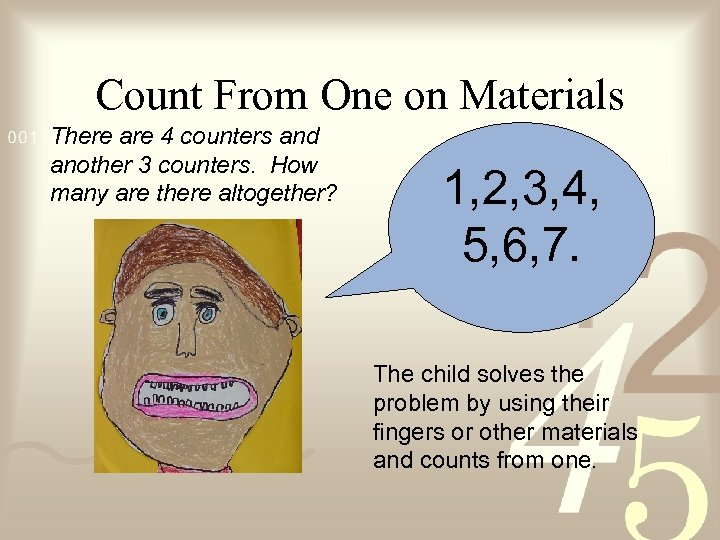Count From One on Materials There are 4 counters and another 3 counters. How many are there altogether? 1, 2, 3, 4, 5, 6, 7. The child solves the problem by using their fingers or other materials and counts from one.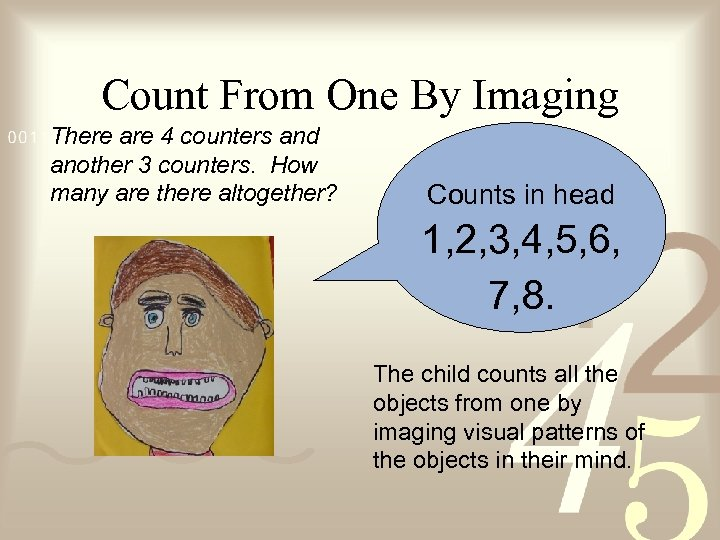Count From One By Imaging There are 4 counters and another 3 counters. How many are there altogether? Counts in head 1, 2, 3, 4, 5, 6, 7, 8. The child counts all the objects from one by imaging visual patterns of the objects in their mind.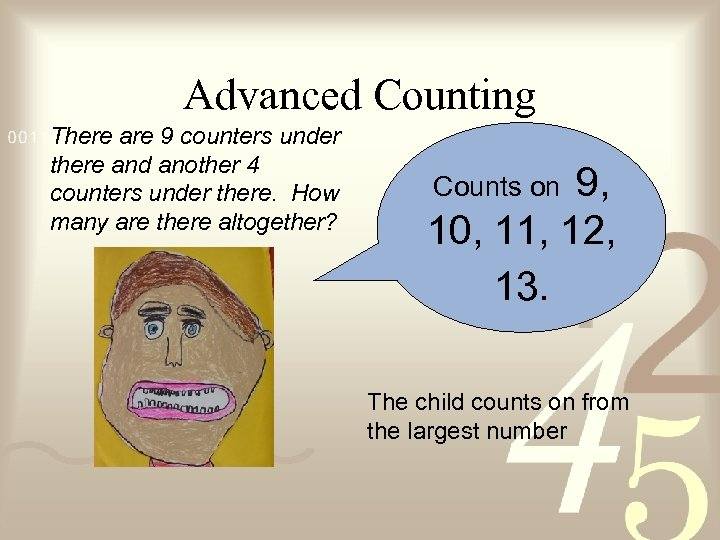Advanced Counting There are 9 counters under there and another 4 counters under there. How many are there altogether? 9, 10, 11, 12, 13. Counts on The child counts on from the largest number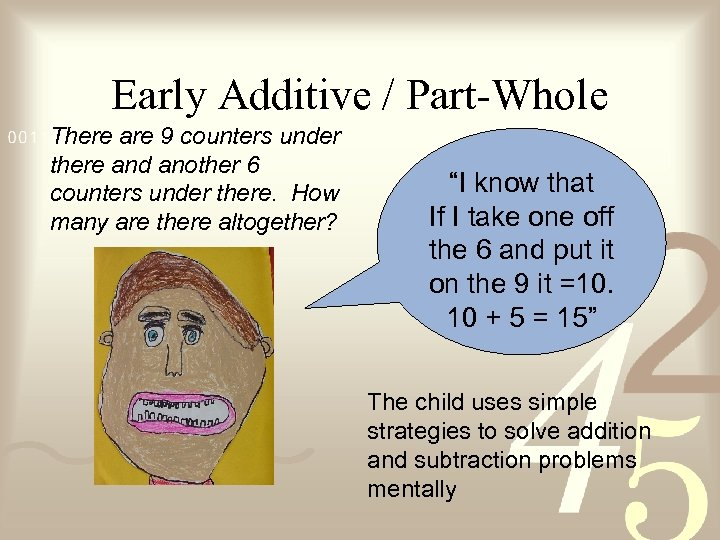Early Additive / Part-Whole There are 9 counters under there and another 6 counters under there. How many are there altogether? “I know that If I take one off the 6 and put it on the 9 it =10. 10 + 5 = 15” The child uses simple strategies to solve addition and subtraction problems mentally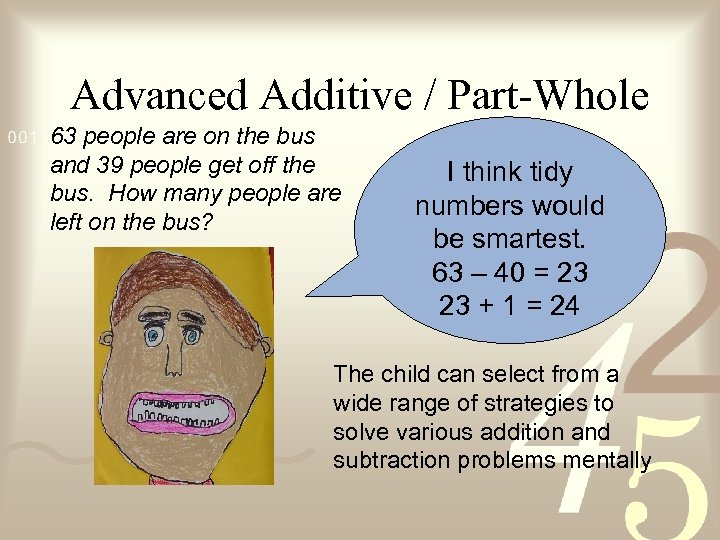Advanced Additive / Part-Whole 63 people are on the bus and 39 people get off the bus. How many people are left on the bus? I think tidy numbers would be smartest. 63 – 40 = 23 23 + 1 = 24 The child can select from a wide range of strategies to solve various addition and subtraction problems mentally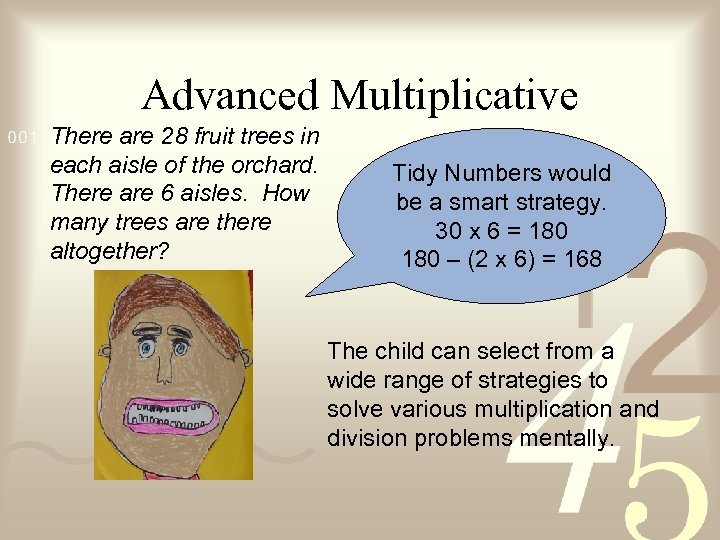Advanced Multiplicative There are 28 fruit trees in each aisle of the orchard. There are 6 aisles. How many trees are there altogether? Tidy Numbers would be a smart strategy. 30 x 6 = 180 – (2 x 6) = 168 The child can select from a wide range of strategies to solve various multiplication and division problems mentally.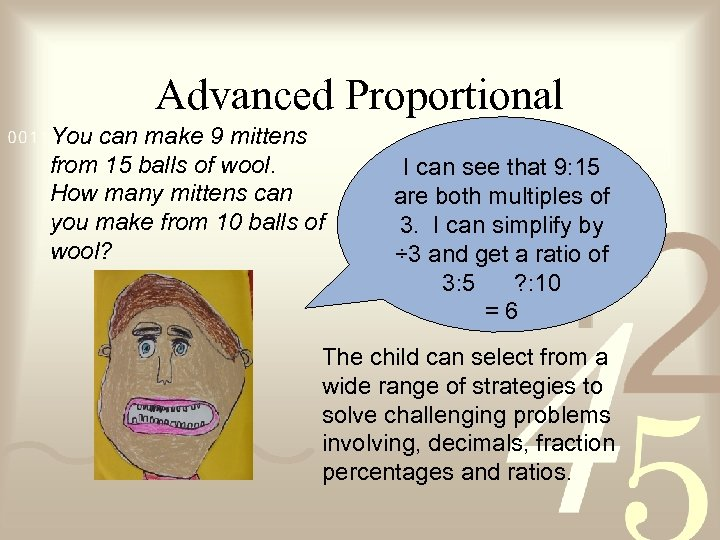Advanced Proportional You can make 9 mittens from 15 balls of wool. How many mittens can you make from 10 balls of wool? I can see that 9: 15 are both multiples of 3. I can simplify by ÷ 3 and get a ratio of 3: 5 ? : 10 =6 The child can select from a wide range of strategies to solve challenging problems involving, decimals, fraction percentages and ratios.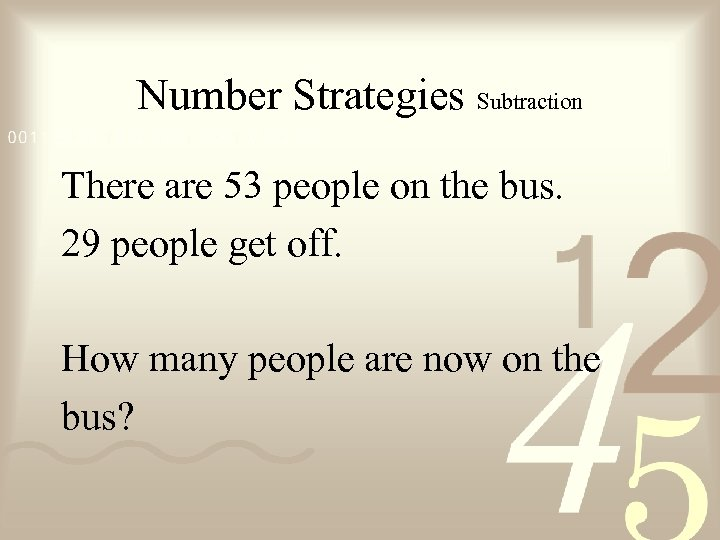Number Strategies Subtraction There are 53 people on the bus. 29 people get off. How many people are now on the bus?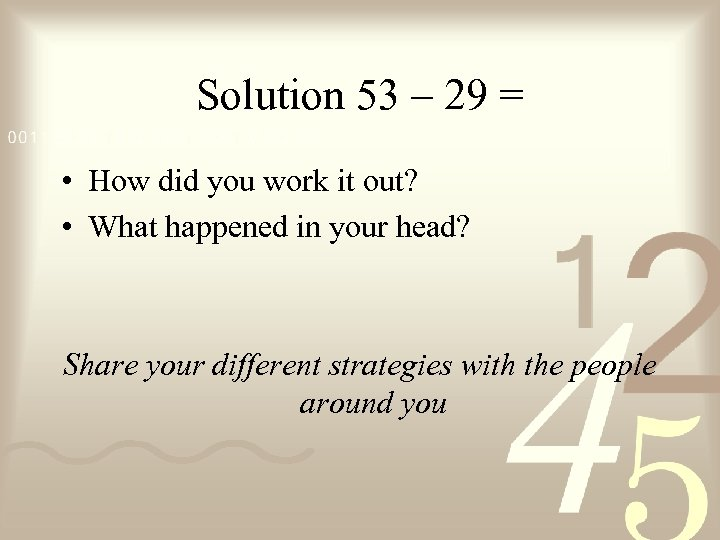Solution 53 – 29 = • How did you work it out? • What happened in your head? Share your different strategies with the people around you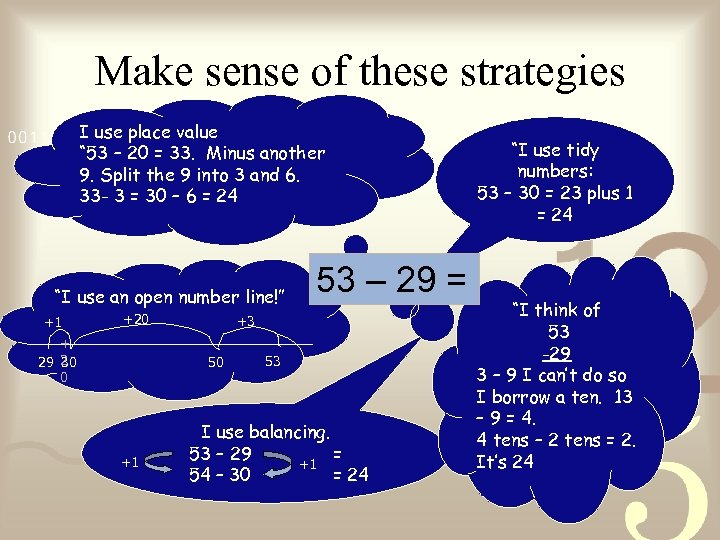Make sense of these strategies I use place value “ 53 – 20 = 33. Minus another 9. Split the 9 into 3 and 6. 33 - 3 = 30 – 6 = 24 “I use an open number line!” +1 +20 + 30 29 2 0 +3 50 +1 53 – 29 = 53 I use balancing. 53 – 29 = +1 54 – 30 = 24 “I use tidy numbers: 53 – 30 = 23 plus 1 = 24 “I think of 53 -29 3 – 9 I can’t do so I borrow a ten. 13 – 9 = 4. 4 tens – 2 tens = 2. It’s 24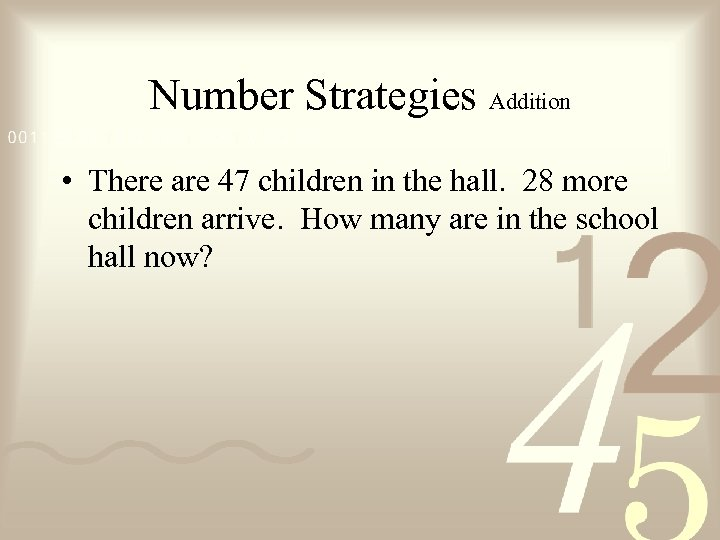Number Strategies Addition • There are 47 children in the hall. 28 more children arrive. How many are in the school hall now?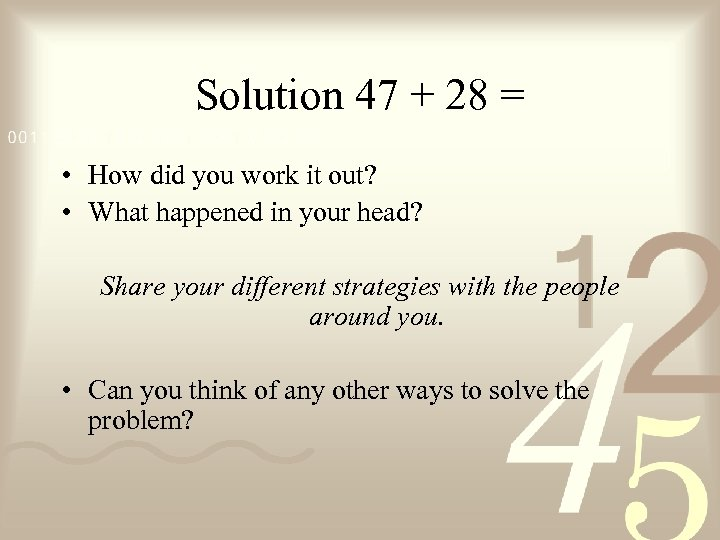Solution 47 + 28 = • How did you work it out? • What happened in your head? Share your different strategies with the people around you. • Can you think of any other ways to solve the problem?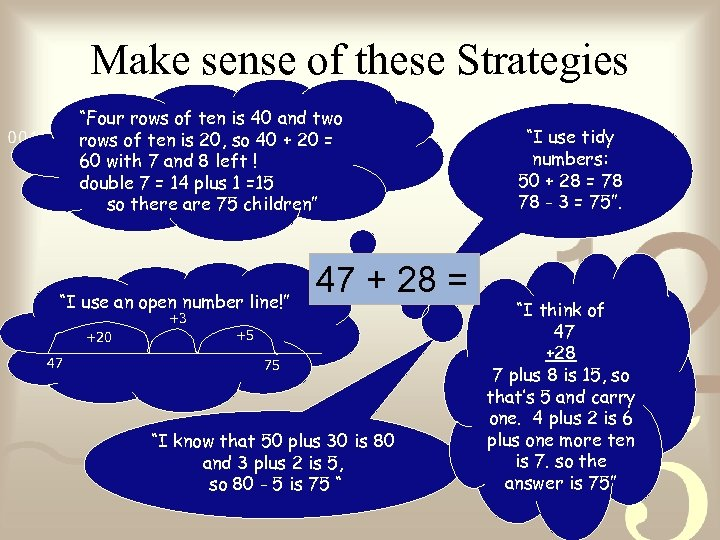Make sense of these Strategies “Four rows of ten is 40 and two rows of ten is 20, so 40 + 20 = 60 with 7 and 8 left ! double 7 = 14 plus 1 =15 so there are 75 children” “I use an open number line!” +3 +20 47 47 + 28 = +5 75 “I know that 50 plus 30 is 80 and 3 plus 2 is 5, so 80 - 5 is 75 “ “I use tidy numbers: 50 + 28 = 78 78 - 3 = 75”. “I think of 47 +28 7 plus 8 is 15, so that’s 5 and carry one. 4 plus 2 is 6 plus one more ten is 7. so the answer is 75”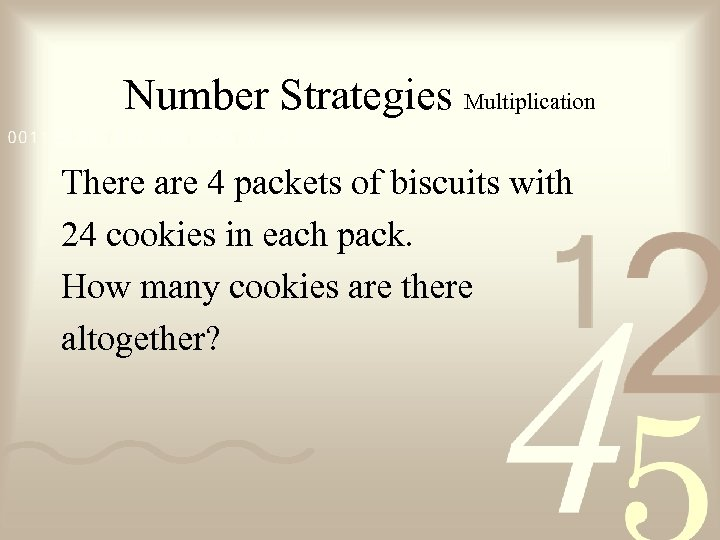Number Strategies Multiplication There are 4 packets of biscuits with 24 cookies in each pack. How many cookies are there altogether?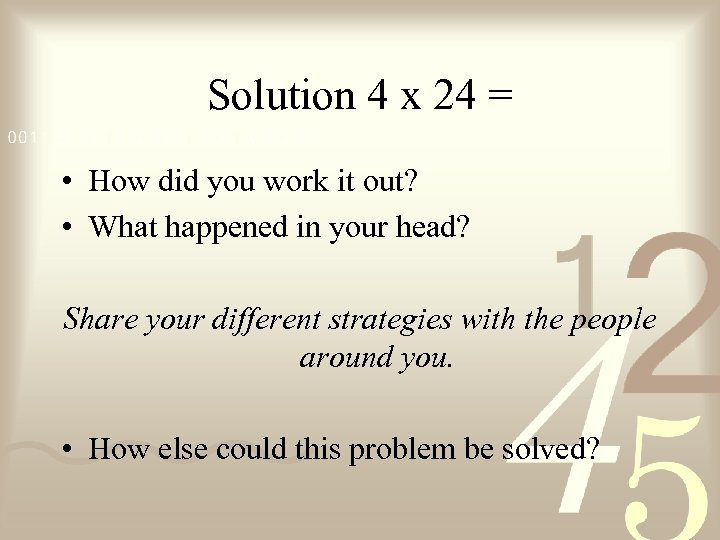Solution 4 x 24 = • How did you work it out? • What happened in your head? Share your different strategies with the people around you. • How else could this problem be solved?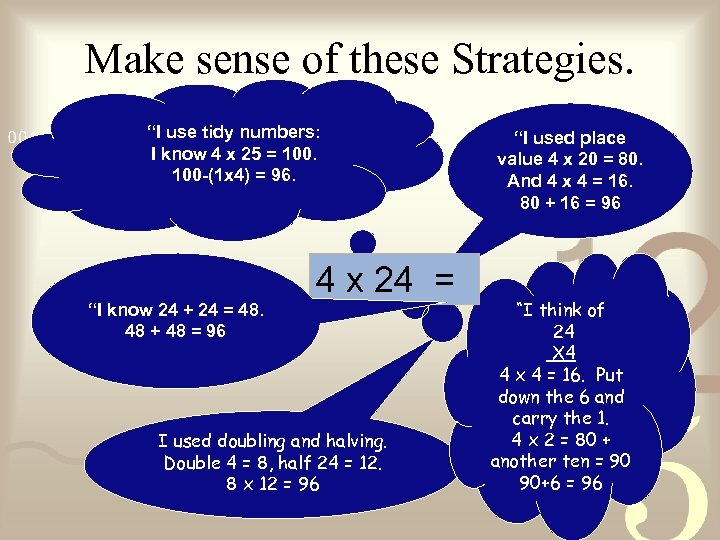Make sense of these Strategies. “I use tidy numbers: I know 4 x 25 = 100 -(1 x 4) = 96. 4 x 24 = “I know 24 + 24 = 48. 48 + 48 = 96 I used doubling and halving. Double 4 = 8, half 24 = 12. 8 x 12 = 96 “I used place value 4 x 20 = 80. And 4 x 4 = 16. 80 + 16 = 96 “I think of 24 X 4 4 x 4 = 16. Put down the 6 and carry the 1. 4 x 2 = 80 + another ten = 90 90+6 = 96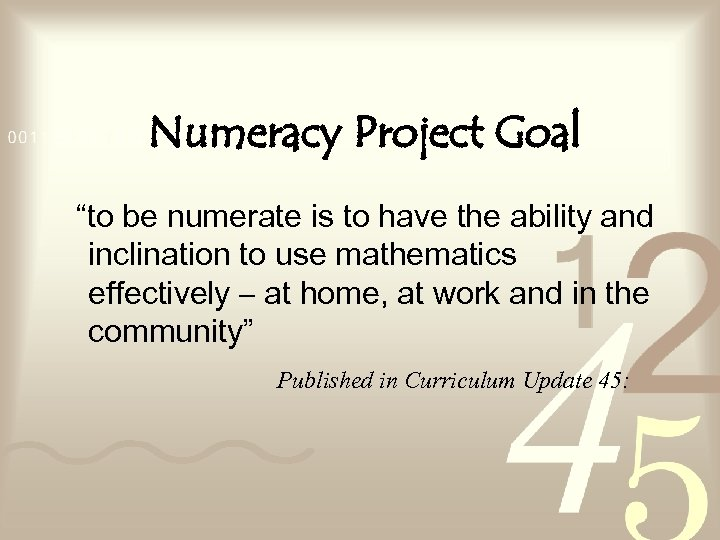Numeracy Project Goal “to be numerate is to have the ability and inclination to use mathematics effectively – at home, at work and in the community” Published in Curriculum Update 45: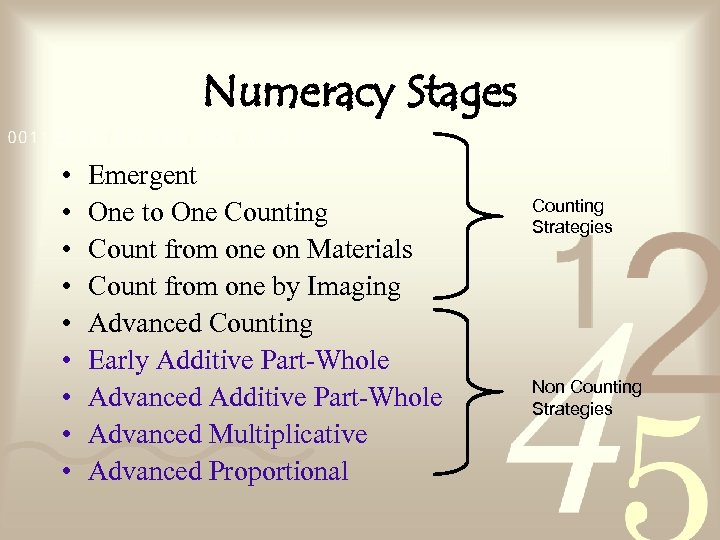Numeracy Stages • • • Emergent One to One Counting Count from one on Materials Count from one by Imaging Advanced Counting Early Additive Part-Whole Advanced Multiplicative Advanced Proportional Counting Strategies Non Counting Strategies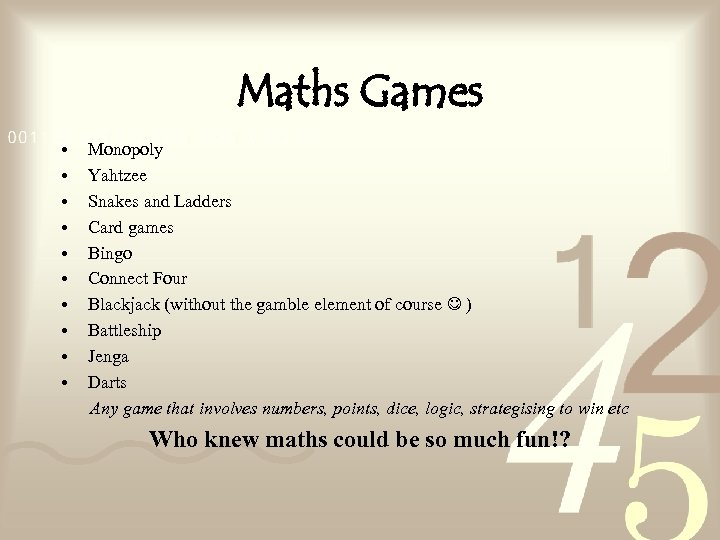Maths Games • • • Monopoly Yahtzee Snakes and Ladders Card games Bingo Connect Four Blackjack (without the gamble element of course ) Battleship Jenga Darts Any game that involves numbers, points, dice, logic, strategising to win etc Who knew maths could be so much fun!?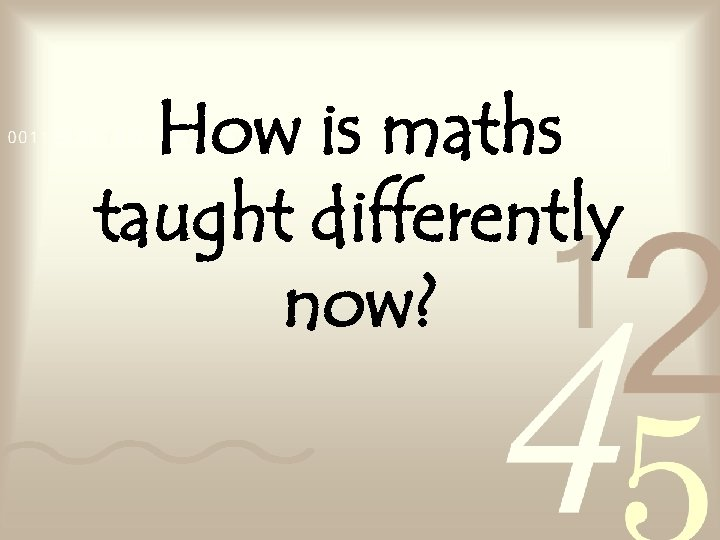How is maths taught differently now?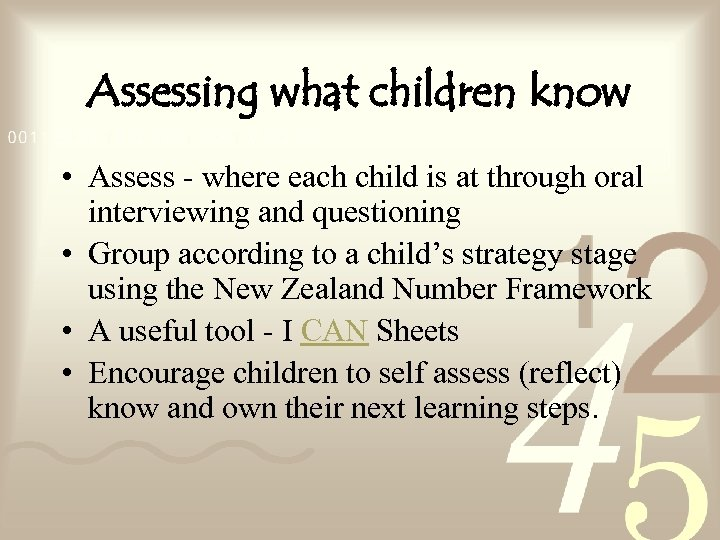Assessing what children know • Assess - where each child is at through oral interviewing and questioning • Group according to a child’s strategy stage using the New Zealand Number Framework • A useful tool - I CAN Sheets • Encourage children to self assess (reflect) know and own their next learning steps.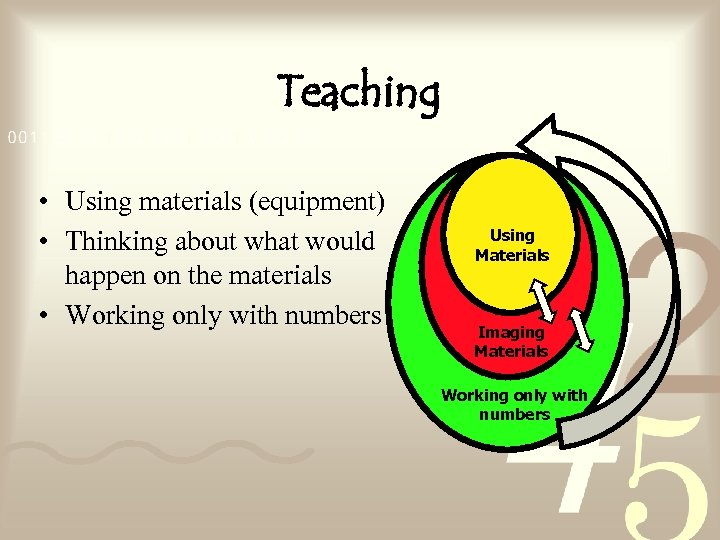Teaching • Using materials (equipment) • Thinking about what would happen on the materials • Working only with numbers Using Materials Imaging Materials Working only with numbers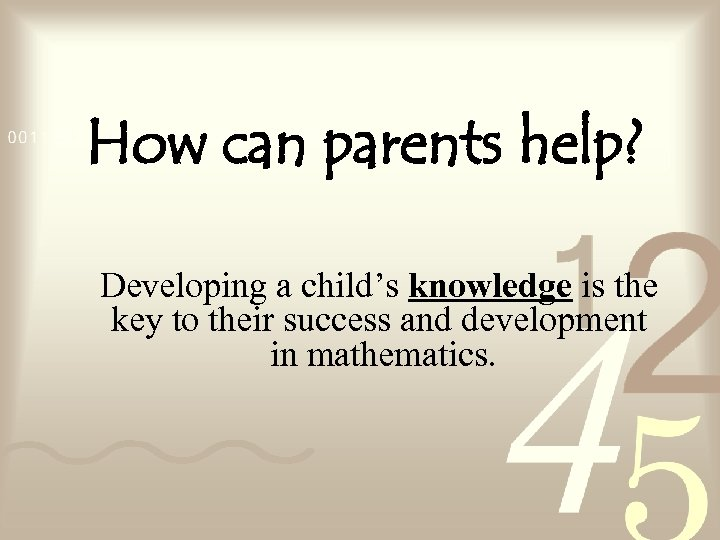How can parents help? Developing a child’s knowledge is the key to their success and development in mathematics.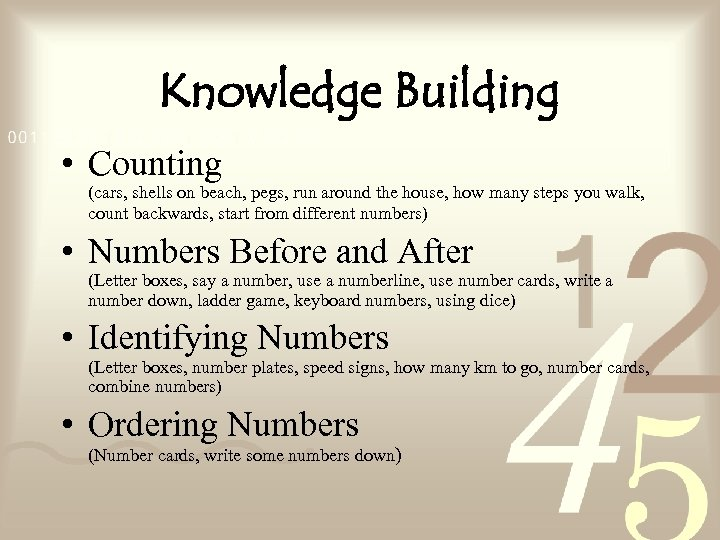Knowledge Building • Counting (cars, shells on beach, pegs, run around the house, how many steps you walk, count backwards, start from different numbers) • Numbers Before and After (Letter boxes, say a number, use a numberline, use number cards, write a number down, ladder game, keyboard numbers, using dice) • Identifying Numbers (Letter boxes, number plates, speed signs, how many km to go, number cards, combine numbers) • Ordering Numbers (Number cards, write some numbers down)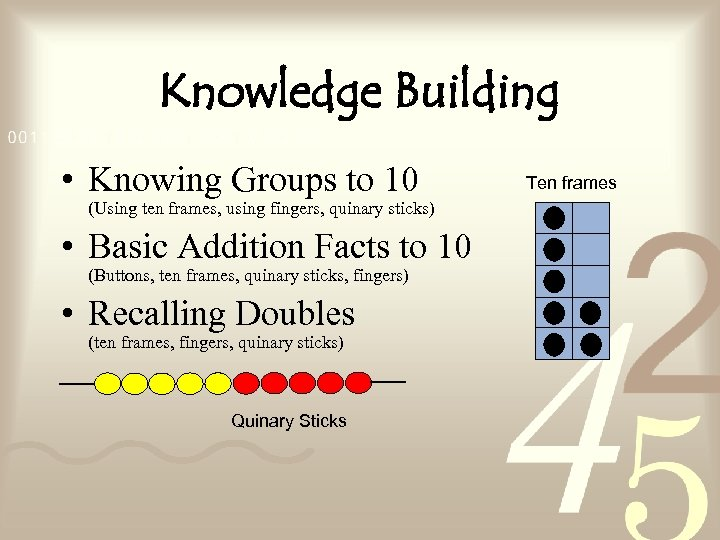Knowledge Building • Knowing Groups to 10 (Using ten frames, using fingers, quinary sticks) • Basic Addition Facts to 10 (Buttons, ten frames, quinary sticks, fingers) • Recalling Doubles (ten frames, fingers, quinary sticks) Quinary Sticks Ten frames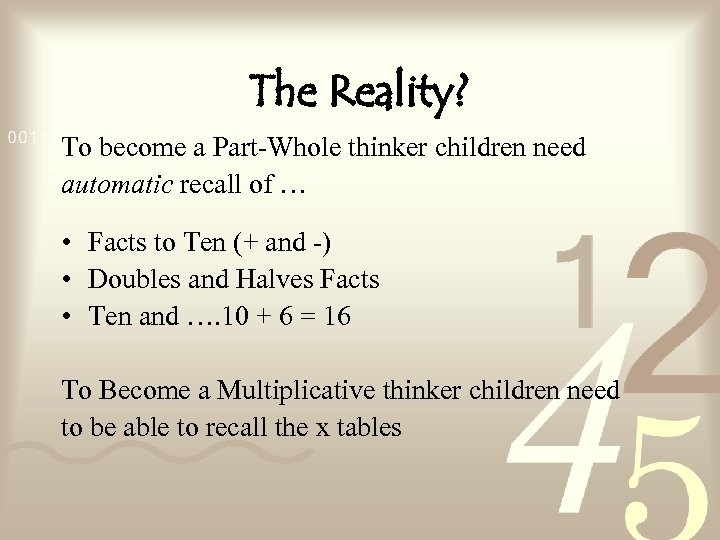The Reality? To become a Part-Whole thinker children need automatic recall of … • Facts to Ten (+ and -) • Doubles and Halves Facts • Ten and …. 10 + 6 = 16 To Become a Multiplicative thinker children need to be able to recall the x tables